﻿ Math formula stock image. Image of chalk, science, blackboard - 10426145

# Math formula.

## Part of math formula on blackboard

Designers also selected these stock photos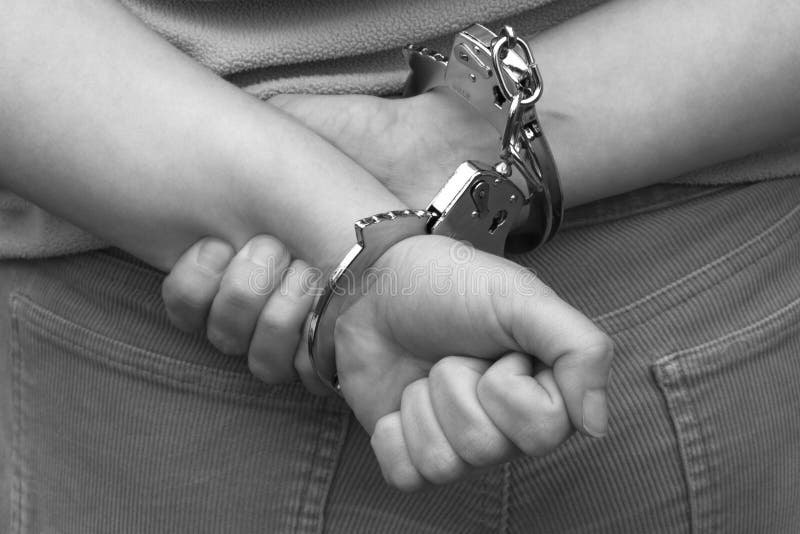Handcuffed individual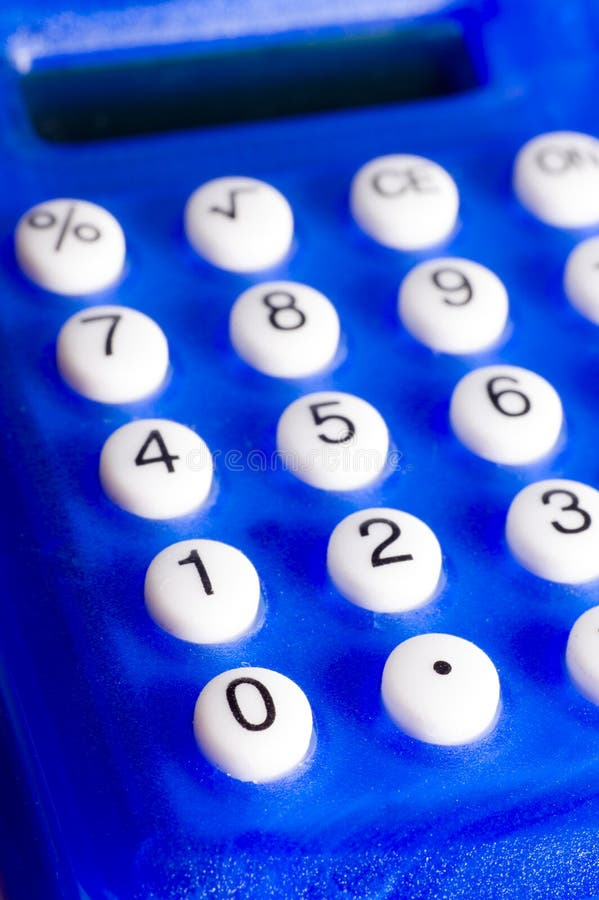Blue calculator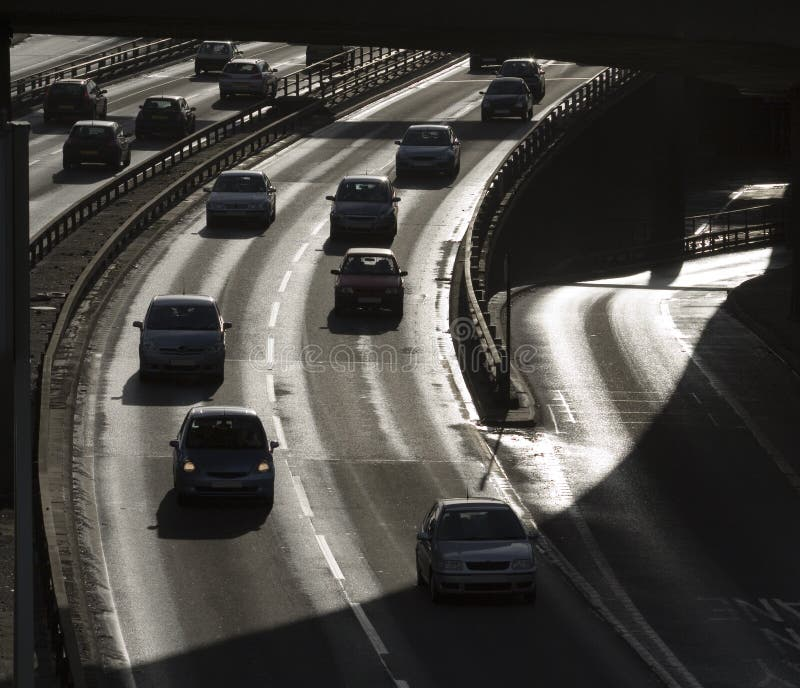Urban trafficManta ray with scuba diver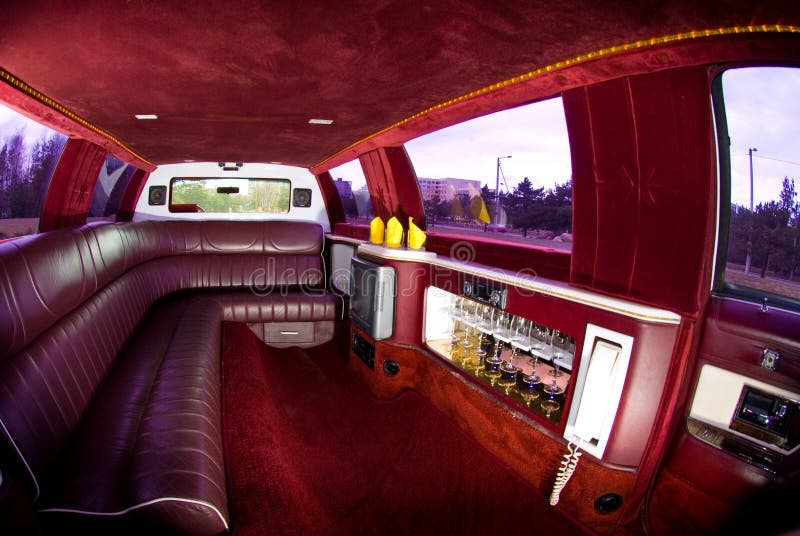Limousine Interior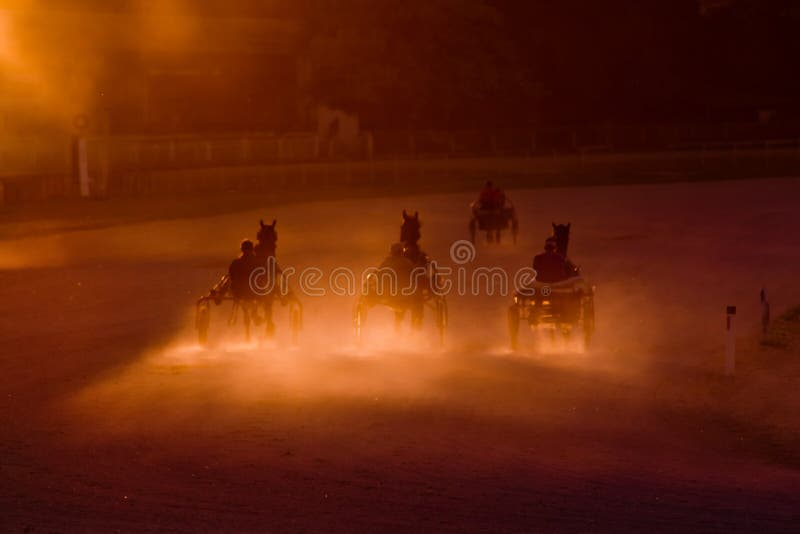Chariots of fire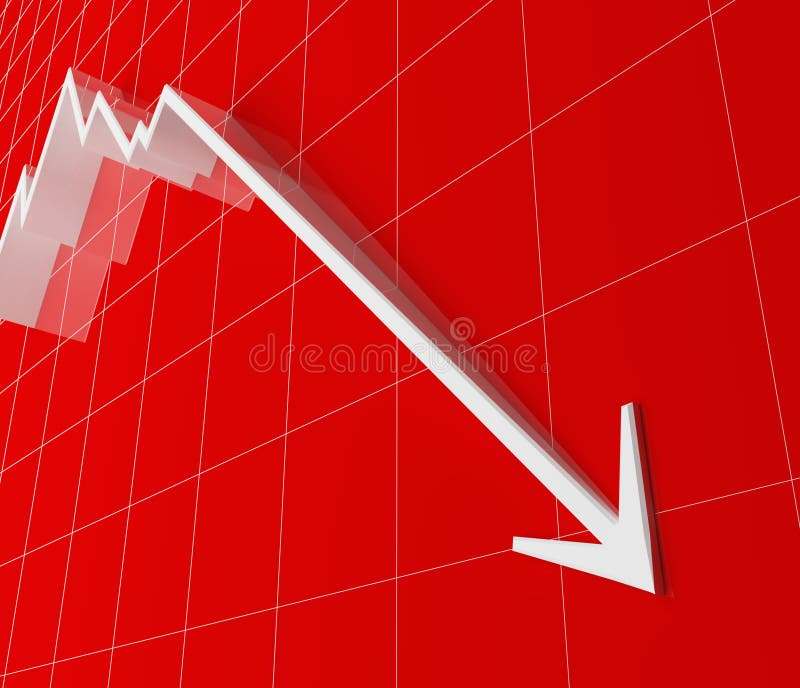Financial stat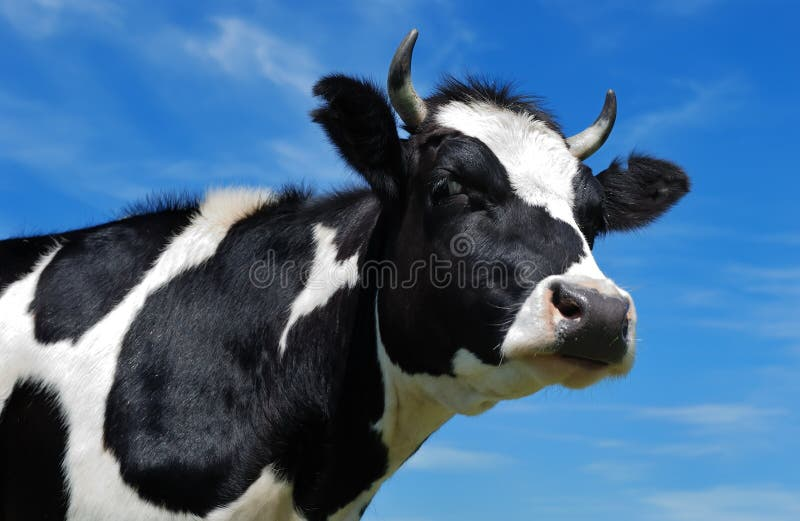Close-up view of horned cow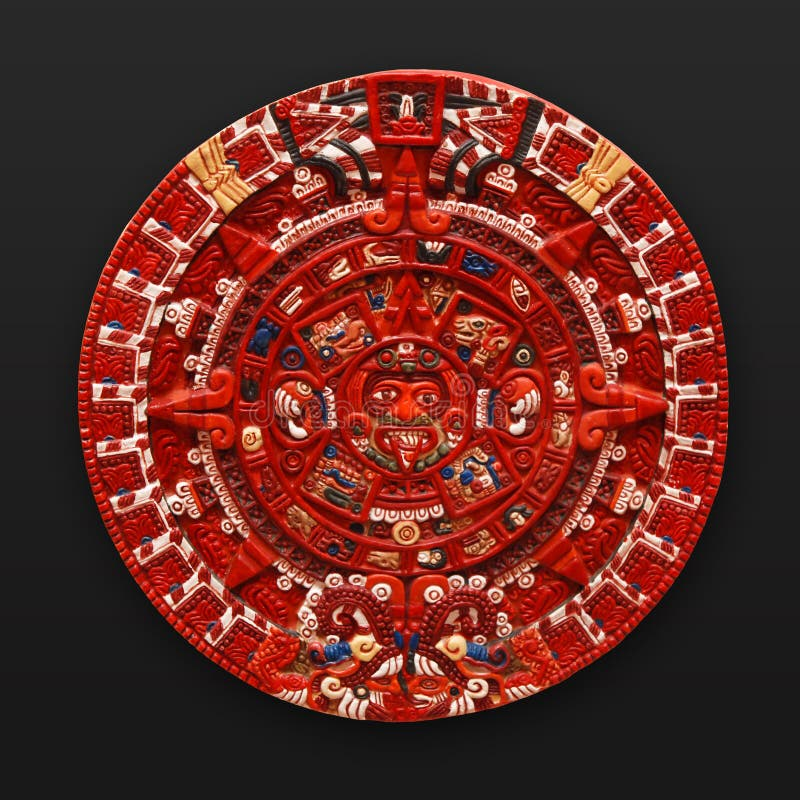Stone aztec calendar latin america
More similar stock images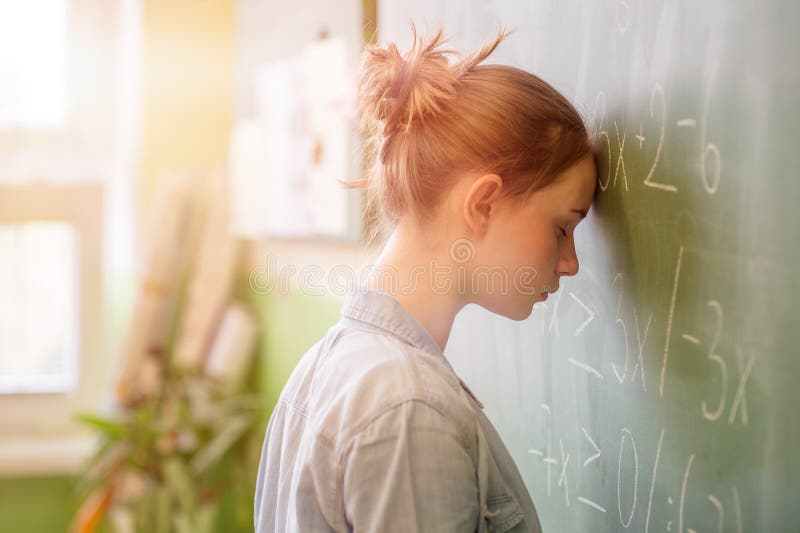Teenager girl in math class overwhelmed by the math formula.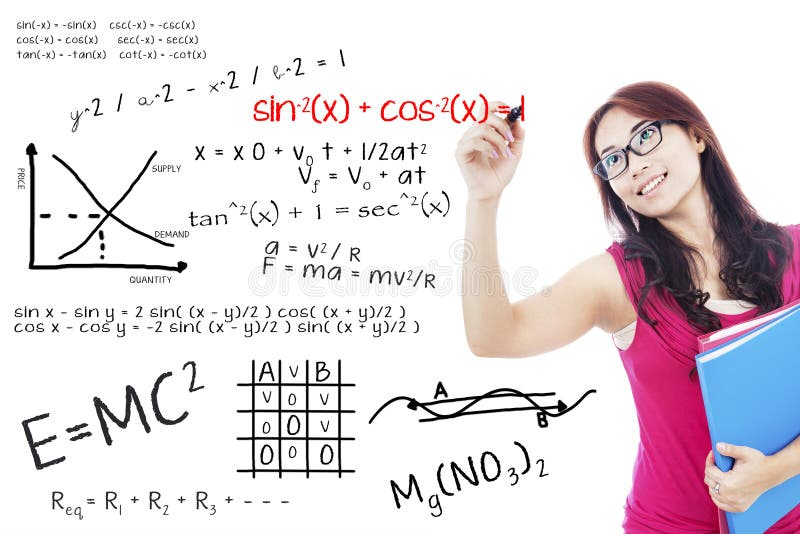College student write math formula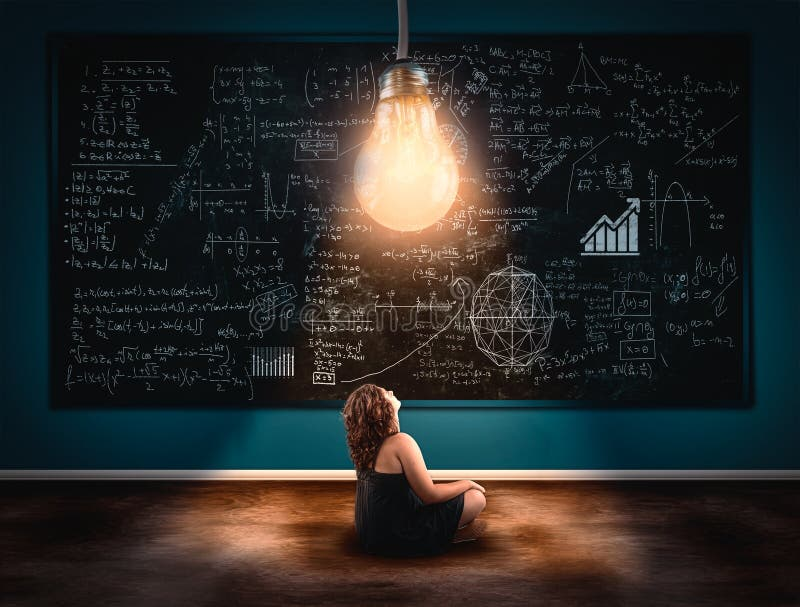The concept of solving a math formula.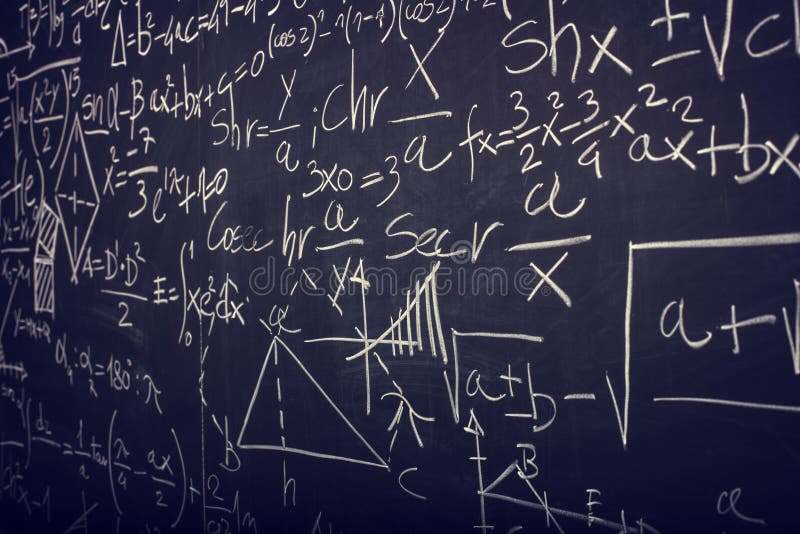Blackboard with math formula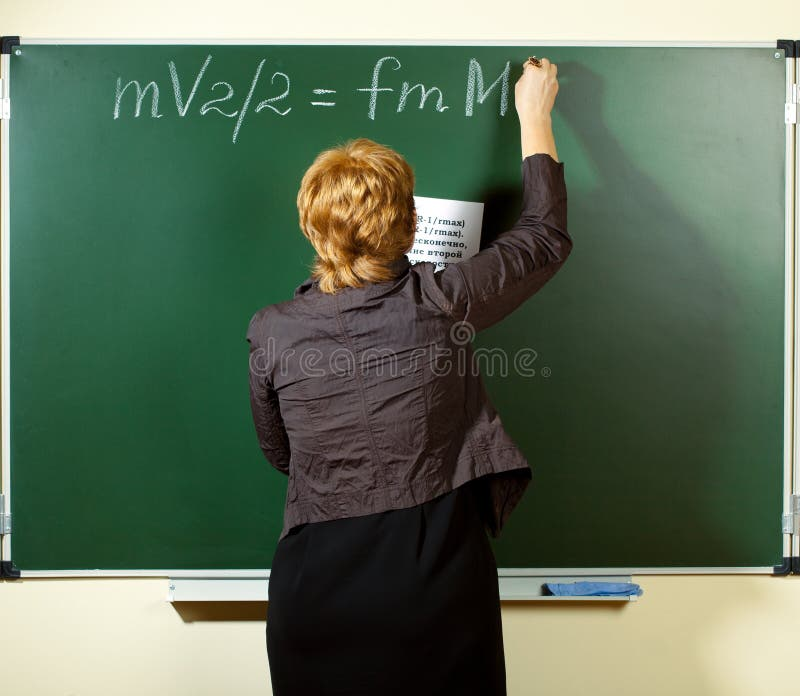Math teacher writing formula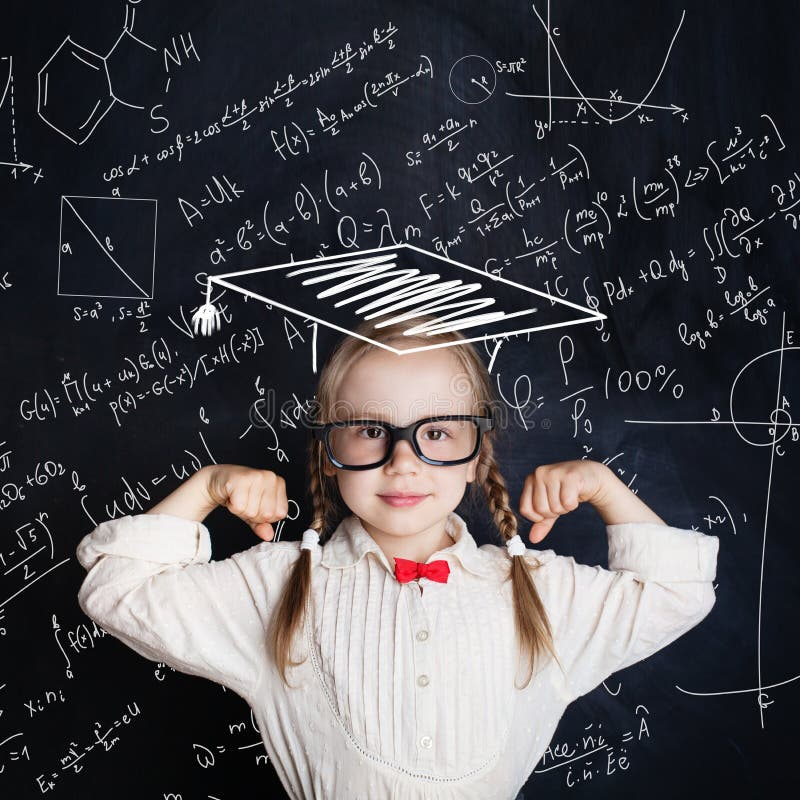Little genius female child on hand drawings math science formula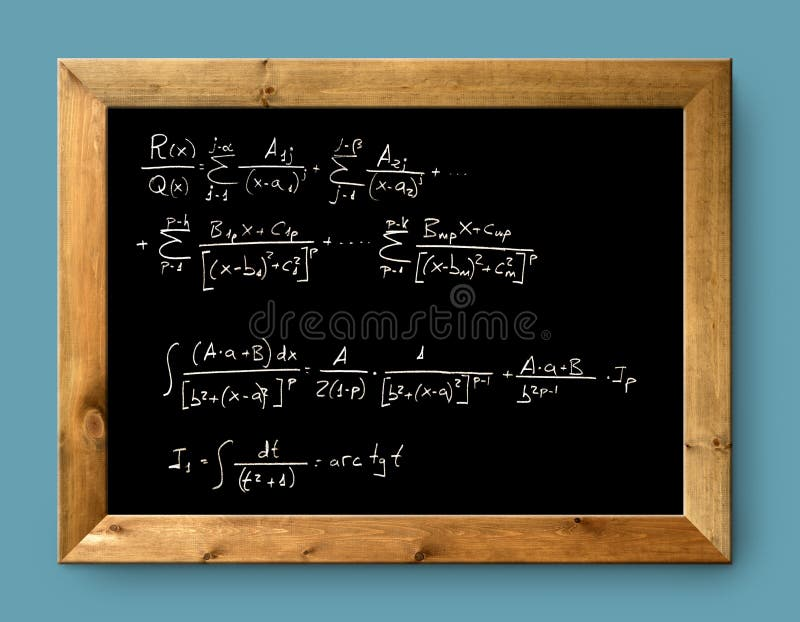Board black blackboard difficult formula math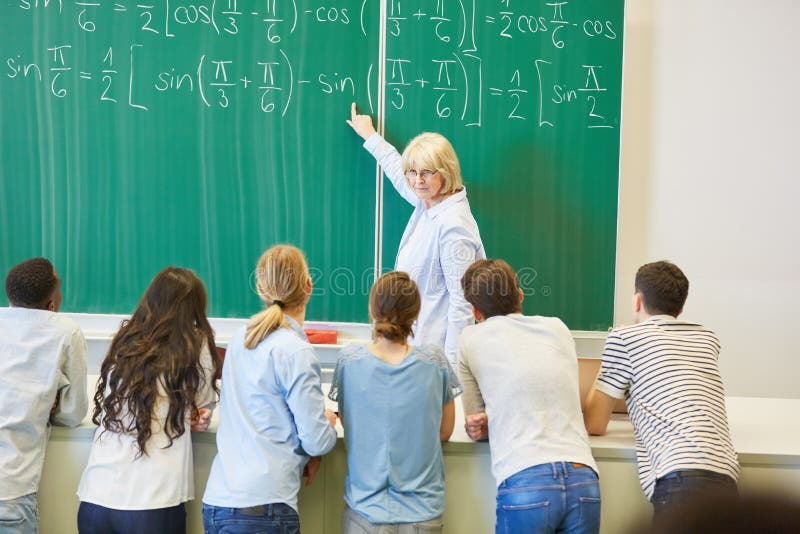Teacher in math class explains formula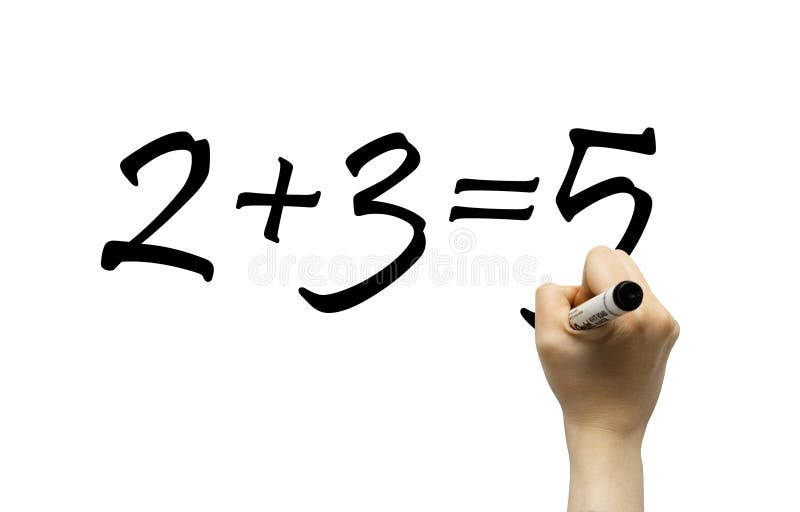Hand writing simple math formula
More stock photos from Inga Ivanova's portfolio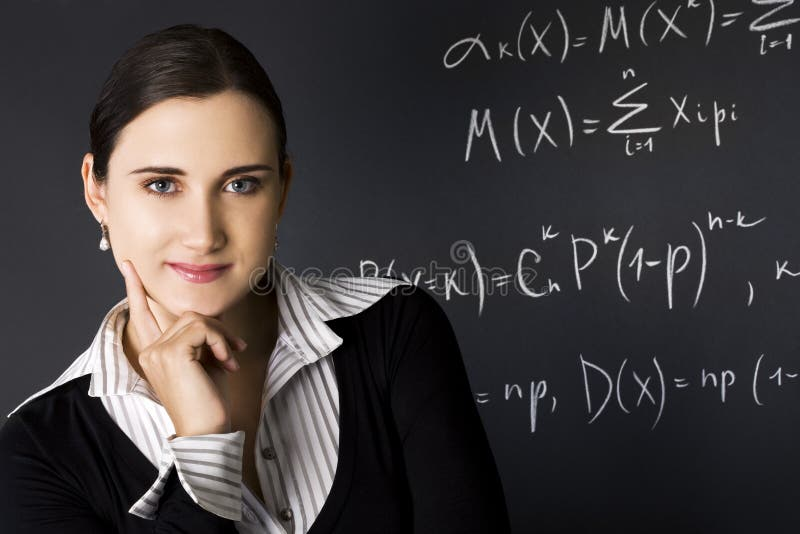Clever mind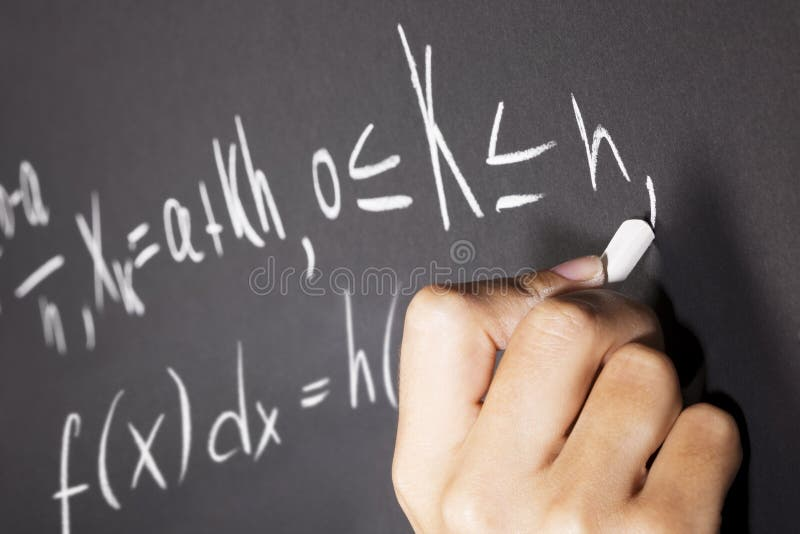Math equations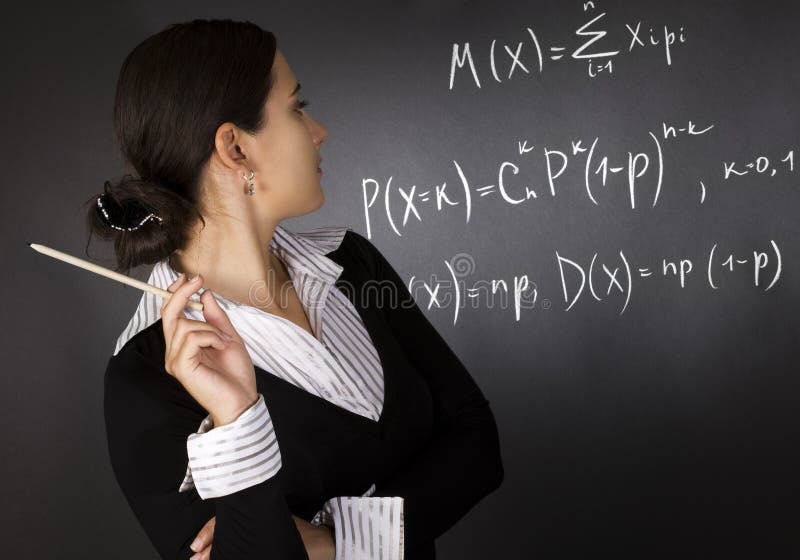Young teacher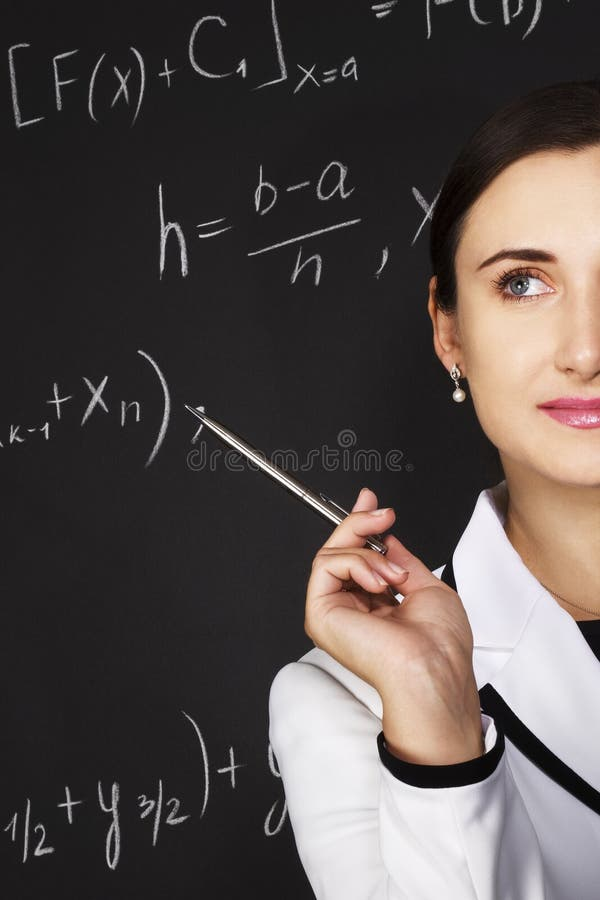Young teacher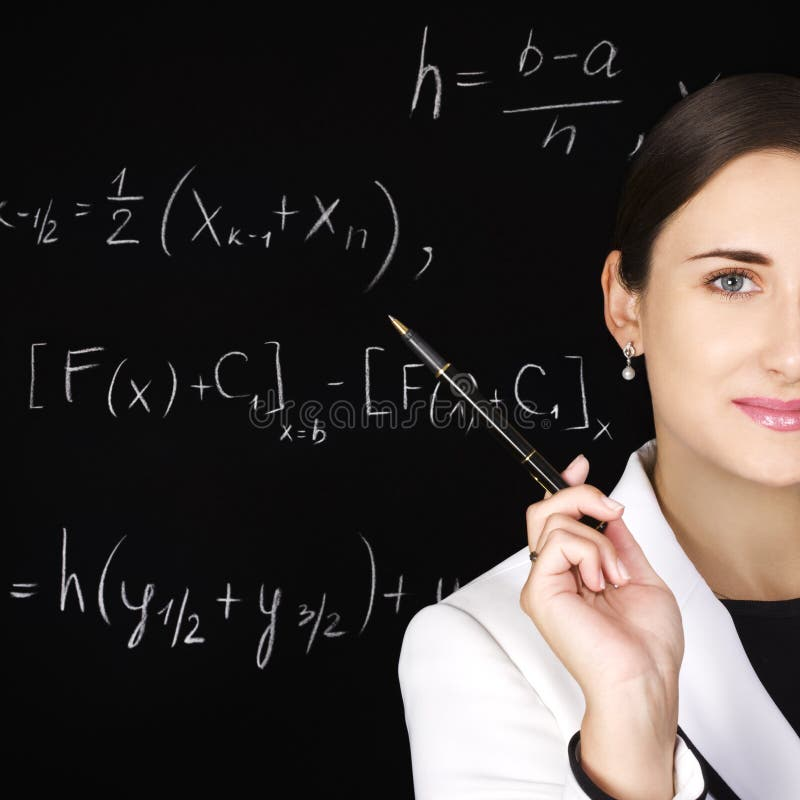Young teacher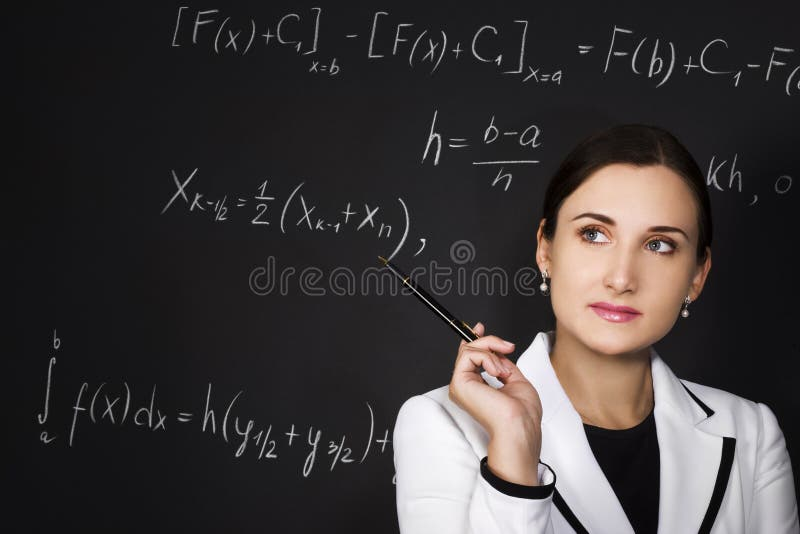Young teacher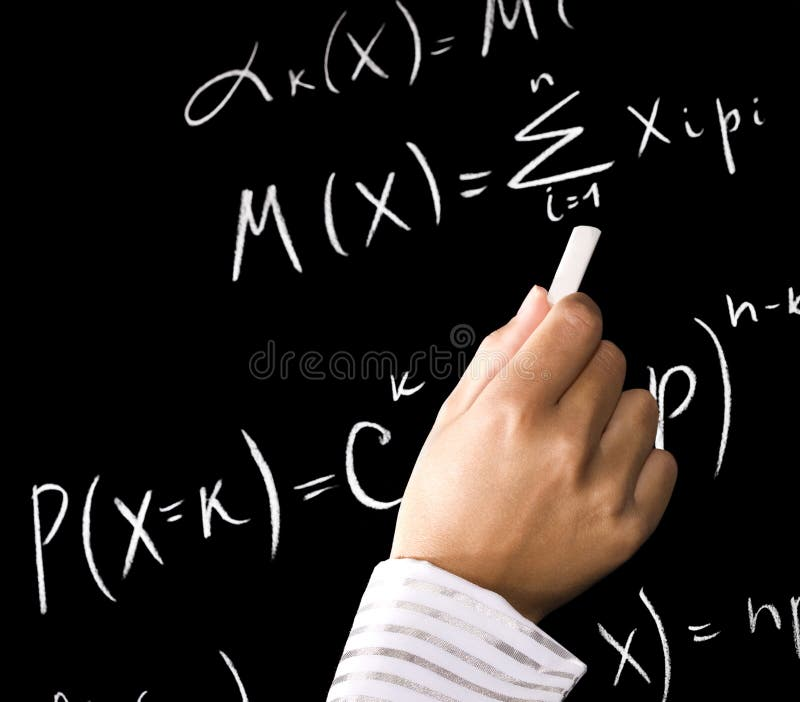Math equations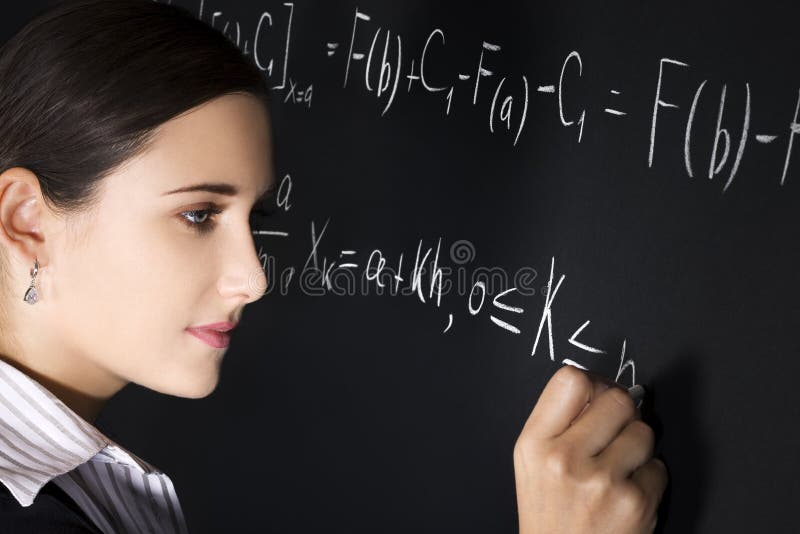Young teacher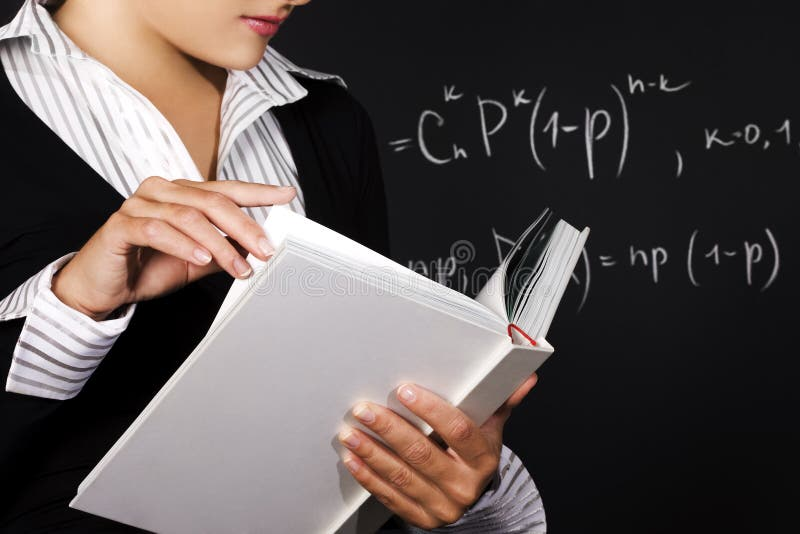Young teacher
Related categories
Extended licenses